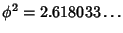## Triangular GraphThe triangular graph withnodes on a side is denoted. Tutte (1970) showed that the Chromatic Polynomials of planar triangular graphs possess a Root close to, whereis the Golden Mean. More precisely, ifis the number of Vertices of, then(Le Lionnais 1983, p. 46). Every planar triangular graph possesses a Vertex of degree 3, 4, or 5 (Le Lionnais 1983, pp. 49 and 53).

Tutte, W. T. On Chromatic Polynomials and the Golden Ratio.'' J. Combin. Theory 9, 289-296, 1970.